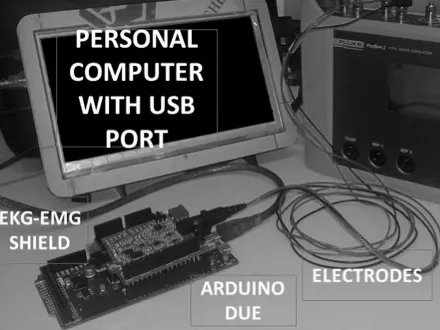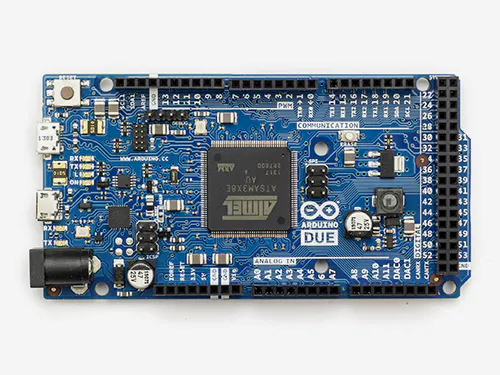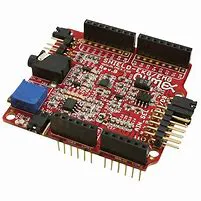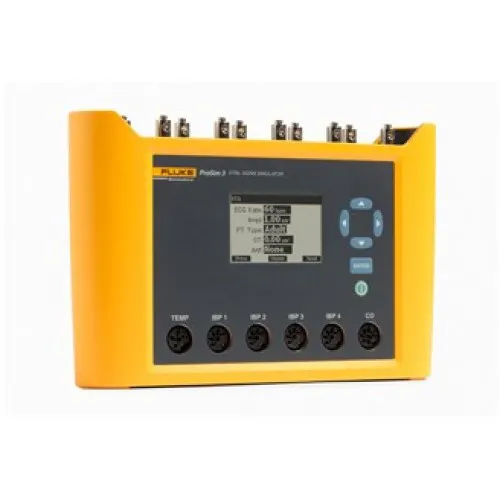# Heart Rate Estimation Using Arduino DUE

This work the design and implementation of a digital system based on open hardware development board applied to heart rate estimation.## Things used in this project

### Hardware componentsArduino Due
×1

## Custom parts and enclosures

### ECG SHIEDL olimex

This is the ECG shield from OLIMEX used as analog amplifier### Patien Simulator

This was used as ECG signal generator. This is profesional tool for testing hear estimation systems## Code

### Complete Program

C/C++
This is the program for heart rate estimation.
```#include <SPI.h>
#include <SD.h>
const int chipSelect = 4;
int N=3000;
int n=0;
int muestra;
int x={0};
int x1={0};
int h={0};
int d={0};
int xr;
int M0;
int M1;
int i;
int c;
int L;
int a=0;
int u=0;
int s=0;
int ini=0;
int m=0;
int temp;
int z=0;
int r=0;
int p=0;

void setup()
{
L=32;
Serial.begin(9600);
Serial.print("Initializing SD card...");
// see if the card is present and can be initialized:
if (!SD.begin(chipSelect))
{
Serial.println("Card failed, or not present");
return;
}
Serial.println("card initialized.");

//////////////////

for(n=0;n<N;n++)
{
delay(1);
}

for (n=L;n<N;n++)
{
c=0;
for (i=0;i<L;i++)
{
c=c+x[n-i];
}
x1[n]=c >> 4;
}

x=0;

for (n=1;n<N;n++)
{
c=x1[n]-x1[n-1];
if (c < 0) c=0;
if (c > 1024) c=0;
x[n]=c;
}

/////////////

for(n=0;n<N;n++)
{
File dataFile = SD.open("ELECTRO.txt", FILE_WRITE);
String dataString = "";
muestra = x[n];
h[muestra]=h[muestra]+1;
dataString += String(muestra);
dataFile.println(dataString);
dataFile.close();
}

for(n=0;n<1100;n++)
{
File dataFile = SD.open("HISTO.txt", FILE_WRITE);
String dataString = "";
muestra = h[n];
dataString += String(muestra);
dataFile.println(dataString);
dataFile.close();
}

d=0;
for (n=1;n<1100;n++)

{
File dataFile = SD.open("DERIV.txt", FILE_WRITE);
String dataString = "";
d[n]=h[n]-h[n-1];
dataString = String(d[n]);
dataFile.println(dataString);
dataFile.close();
}

c=0;
p=0;
for (n=1;n<1100;n++)
{
if (h[n]>c)
{
c= h[n];
p=n;
}
}

for (n=p;n<1100;n++)
{
if (h[n]==0)
{
c= n;
break;
}
}

Serial.println("Valor Umbral");
Serial.println(c);

for (n=0;n<N;n++)
{
File dataFile = SD.open("UMBRAL.txt", FILE_WRITE);
String dataString = "";
if (x[n]>c)
{
x[n]=1;
}
else
{
x[n]=0;
}
dataString = String(x[n]);
dataFile.println(dataString);
dataFile.close();
}

for (n=0;n<N;n++)
{

if (x[n]==1)
{
ini=n;
break;
}

}

Serial.println(ini);

for (m=ini;m<N;m++)
{
if (x[m]==0)
{
temp=temp+1;
z=0;
}
else
{
z=z+1;
if (z<=1)
{
r=r+1;
xr[r]=temp;
temp=0;
z=z+1;
}
else
{
z=z+1;
temp=temp+1;
}
}
}

Serial.println(r);

for (n=0;n<10;n++)
{

File dataFile = SD.open("FREC.txt", FILE_WRITE);
String dataString = "";
dataString = String(xr[n]);
dataFile.println(dataString);
dataFile.close();
}

}

void loop() {

}
```

## Credits

### javiergonzalezb

3 projects • 1 follower